# mne.minimum_norm.InverseOperator#

class mne.minimum_norm.InverseOperator[source]#

InverseOperator class to represent info from inverse operator.

Methods

 `__contains__`(key, /) True if the dictionary has the specified key, else False. `__getitem__` x.__getitem__(y) <==> x[y] Implement iter(self). Return len(self). Return a copy of the InverseOperator. `fromkeys`(iterable[, value]) Create a new dictionary with keys from iterable and values set to value. `get`(key[, default]) Return the value for key if key is in the dictionary, else default. `pop`(key[, default]) If the key is not found, return the default if given; otherwise, raise a KeyError. Remove and return a (key, value) pair as a 2-tuple. `setdefault`(key[, default]) Insert key with a value of default if key is not in the dictionary. `update`([E, ]**F) If E is present and has a .keys() method, then does: for k in E: D[k] = E[k] If E is present and lacks a .keys() method, then does: for k, v in E: D[k] = v In either case, this is followed by: for k in F: D[k] = F[k]
__contains__(key, /)#

True if the dictionary has the specified key, else False.

__getitem__()#

x.__getitem__(y) <==> x[y]

__iter__(/)#

Implement iter(self).

__len__(/)#

Return len(self).

clear() None.  Remove all items from D.#
copy()[source]#

Return a copy of the InverseOperator.

fromkeys(iterable, value=None, /)#

Create a new dictionary with keys from iterable and values set to value.

get(key, default=None, /)#

Return the value for key if key is in the dictionary, else default.

items() a set-like object providing a view on D's items#
keys() a set-like object providing a view on D's keys#
pop(key, default=<unrepresentable>, /)#

If the key is not found, return the default if given; otherwise, raise a KeyError.

popitem(/)#

Remove and return a (key, value) pair as a 2-tuple.

Pairs are returned in LIFO (last-in, first-out) order. Raises KeyError if the dict is empty.

setdefault(key, default=None, /)#

Insert key with a value of default if key is not in the dictionary.

Return the value for key if key is in the dictionary, else default.

update([E, ]**F) None.  Update D from dict/iterable E and F.#

If E is present and has a .keys() method, then does: for k in E: D[k] = E[k] If E is present and lacks a .keys() method, then does: for k, v in E: D[k] = v In either case, this is followed by: for k in F: D[k] = F[k]

values() an object providing a view on D's values#

## Examples using `mne.minimum_norm.InverseOperator`#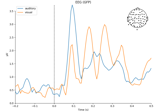Overview of MEG/EEG analysis with MNE-Python

Overview of MEG/EEG analysis with MNE-Python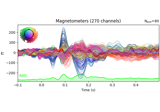Working with CTF data: the Brainstorm auditory dataset

Working with CTF data: the Brainstorm auditory dataset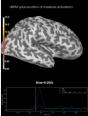Source localization with MNE, dSPM, sLORETA, and eLORETA

Source localization with MNE, dSPM, sLORETA, and eLORETA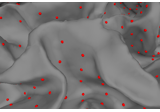The role of dipole orientations in distributed source localization

The role of dipole orientations in distributed source localization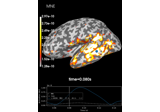Computing various MNE solutions

Computing various MNE solutions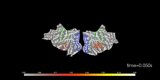Visualize source time courses (stcs)

Visualize source time courses (stcs)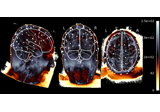EEG source localization given electrode locations on an MRI

EEG source localization given electrode locations on an MRI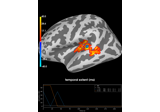Permutation t-test on source data with spatio-temporal clustering

Permutation t-test on source data with spatio-temporal clustering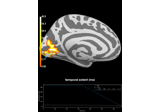Repeated measures ANOVA on source data with spatio-temporal clustering

Repeated measures ANOVA on source data with spatio-temporal clustering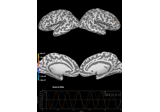Corrupt known signal with point spread

Corrupt known signal with point spread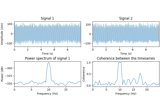DICS for power mapping

DICS for power mapping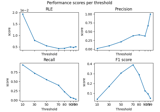Compare simulated and estimated source activity

Compare simulated and estimated source activity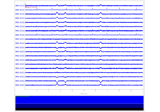Simulate raw data using subject anatomy

Simulate raw data using subject anatomy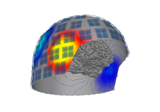Plot the MNE brain and helmet

Plot the MNE brain and helmet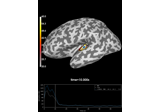Compute Power Spectral Density of inverse solution from single epochs

Compute Power Spectral Density of inverse solution from single epochs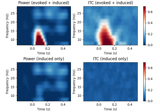Compute power and phase lock in label of the source space

Compute power and phase lock in label of the source space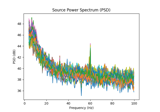Compute source power spectral density (PSD) in a label

Compute source power spectral density (PSD) in a label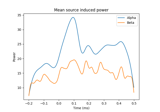Compute induced power in the source space with dSPM

Compute induced power in the source space with dSPM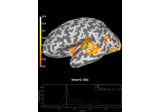Decoding source space data

Decoding source space data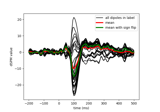Compute MNE-dSPM inverse solution on single epochs

Compute MNE-dSPM inverse solution on single epochs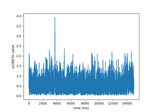Compute sLORETA inverse solution on raw data

Compute sLORETA inverse solution on raw data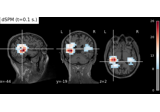Compute MNE-dSPM inverse solution on evoked data in volume source space

Compute MNE-dSPM inverse solution on evoked data in volume source space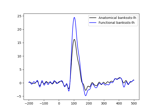Generate a functional label from source estimates

Generate a functional label from source estimates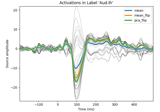Extracting the time series of activations in a label

Extracting the time series of activations in a label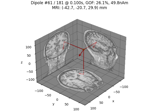Compute sparse inverse solution with mixed norm: MxNE and irMxNE

Compute sparse inverse solution with mixed norm: MxNE and irMxNE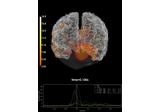Compute MNE inverse solution on evoked data with a mixed source space

Compute MNE inverse solution on evoked data with a mixed source space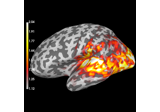Compute source power estimate by projecting the covariance with MNE

Compute source power estimate by projecting the covariance with MNE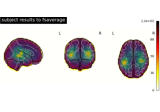Morph volumetric source estimate

Morph volumetric source estimate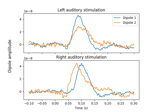Computing source timecourses with an XFit-like multi-dipole model

Computing source timecourses with an XFit-like multi-dipole model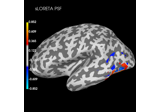Plot point-spread functions (PSFs) and cross-talk functions (CTFs)

Plot point-spread functions (PSFs) and cross-talk functions (CTFs)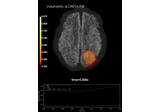Plot point-spread functions (PSFs) for a volume

Plot point-spread functions (PSFs) for a volume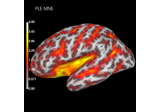Compute spatial resolution metrics in source space

Compute spatial resolution metrics in source space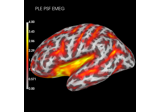Compute spatial resolution metrics to compare MEG with EEG+MEG

Compute spatial resolution metrics to compare MEG with EEG+MEG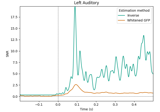Estimate data SNR using an inverse

Estimate data SNR using an inverse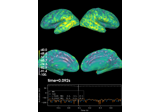Computing source space SNR

Computing source space SNR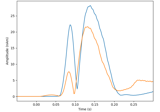Compute MxNE with time-frequency sparse prior

Compute MxNE with time-frequency sparse prior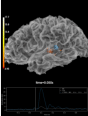Plotting the full vector-valued MNE solution

Plotting the full vector-valued MNE solution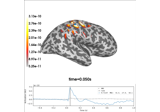Optically pumped magnetometer (OPM) data

Optically pumped magnetometer (OPM) data